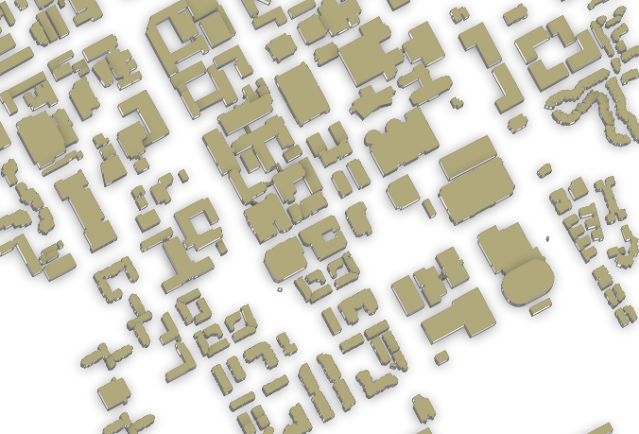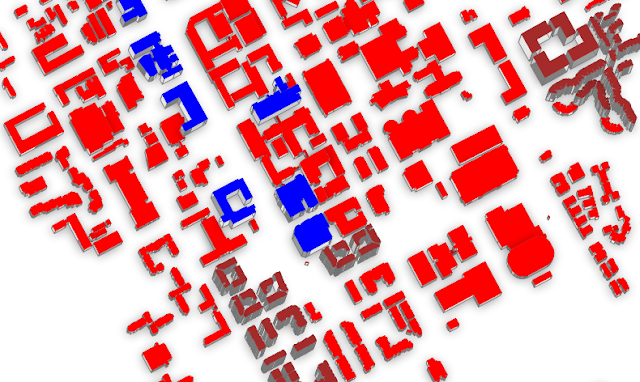# PyQGIS Tutorial: 2.5D Vector Data Rendering

Rendering is an action to draw a data (usually geometric data which consists of point, line and polygon) both 2D and 3D by means of a computer program. It occurs when we want to view a data on a screen. In simple way when a data is added to a screen for example in QGIS map canvas, the rendering process is taking place to draw the data on the screen.

There are many types of data rendering/visualization can be used in QGIS to get more insight or meaning from a data like graduated, rule based, heatmap, categorized, 3D, etc. In this tutorial we will see how to visualize a data in 2.5 dimension (2.5D) like figure 1 below.Figure 1. 2.5D Data Visualization

To make a 2.5D data visualization is quite simple using Symbology menu in the data properties. Because this is about PyQGIS tutorial, I won't discuss how to do it manually and I'm sure you can make it. But we will see how to do it using Python code.

The code for 2.5D rendering consist of three parts:
1. Define a vector layer.
2. Set 2.5D symbol renderer including it's options/methods.
3. Add the vector data to the map.
Let's get started!

#### Define Vector Layer

2.5D visualization works with vector data. Therefore firstly we need to define a vector layer using QgsVectorLayer class as following code. For this tutorial I used a vector in Geopackage format which is called building_level.gpkg.

 ```1 2``` ```path="/home/data/buildings_level.gpkg" building=QgsVectorLayer(path,"Building","ogr") ```

#### 2.5D Rendering

Next we determine the 2.5D symbol renderer with Qgs25DRenderer class. Set the building vector layer symbol to it and set some methods like roof color and wall color. For rendering we also need the height in map unit and angle of features (0 degree is west and rotates in counter clockwise). It can be defined using QgsExpressionContextUtils class wiht setLayerVariable method as in line 8-10 in the following code.

 ``` 1 2 3 4 5 6 7 8 9 10``` ```#DETERMINE 2.5D RENDERER AND SET SOME OPTIONS render25d=Qgs25DRenderer() building.setRenderer(render25d) render25d.setRoofColor(QColor('brown')) render25d.setWallColor(QColor('white')) #DETERMINE ANGLE AND HEIGHT exp=QgsExpressionContextUtils() exp.setLayerVariable(building,"qgis_25d_angle",70) exp.setLayerVariable(building,"qgis_25d_height",10) ```

In the code I only used two renderer methods (roof and wall color). In fact there are many methods available such as: setShadowColor, shadowEnabled, shadowSpred, startRender, stopRender, and many more. You can find the complete 2.5D renderer methods in PyQGIS Documentation.

After finishing the 2.5D symbol rendering and it's methods, now let visualize it into QGIS map canvas using addMapLayer.

```#VISUALIZE IN MAP CANVAS
```

That's it. After running the code. The building vector layer will be rendered as shown in figure 1.

#### 2.5D Multi Layers Rendering

We had discussed how to make 2.5D rendering with a single layer. In this section we will see how to render multiple layers. In this case, I'd like to render different roof color and height for building based on it's level that stored in attribute table as seen in figure 2. Each building's level is multiplied by 10, thus level 1 is 10 in map unit, level 2 is 20 and so forth.Figure 2. Vector Layer Attribute Table

Rendering process takes place for a layer (a group of features). Because of that we need to filter buildings based on it's level. The filtering can be done using a Structured Query Language (SQL) syntax as follow:

SELECT 'buildings_level'.'geom', 'buildings_level'.'building:levels' FROM 'buildings_level' WHERE 'buildings_level'.'building:levels=??

The building level starts from 1 to 3. For that we need to make a loop for filtering each level, set 2.5D symbol renderer, determine it's roof color including height and add it into QGIS map canvas.

Below is the code for multiple 2.5D layers rendering.
 ``` 1 2 3 4 5 6 7 8 9 10 11 12 13 14 15 16 17 18 19 20 21 22 23 24 25 26 27``` ```#DEFINE FILE PATH AND SQL FILTER path="/home/data/buildings_level.gpkg" subset="subset=SELECT 'buildings_level'.'geom', 'buildings_level'.'building:levels' FROM 'buildings_level' WHERE 'buildings_level'.'building:levels'" levels=[1,2,3] #Building level color=['red','blue','brown'] #Roof color. Level 1=red. 2=blue. 3=brown i=0 exp=QgsExpressionContextUtils() #LOOP for level in levels: #CONSTRUCT SQL QUERY query=path+"|"+subset+"="+str(level) layer=QgsVectorLayer(query,"level "+str(level)) i +=1 #DEFINE 2.5D RENDERER r=Qgs25DRenderer() layer.setRenderer(r) #2.5D METHOD/OPTIONS exp.setLayerVariable(layer,"qgis_25d_angle",70) #Set Angle height=level*10 #Set Height exp.setLayerVariable(layer,"qgis_25d_height",height) r.setRoofColor(QColor(color[i-1])) #Set Color #ADD TO QGIS QgsProject.instance().addMapLayer(layer) ```

After executed the code, the building will be rendered in different roof color and height as seen in figure 3.Figure 3. 2.5D Multiple Layers Rendering

That's all this tutorial how to make 2.5D data rendering/visualization for vector layer. Hope this tutorial could help you in learning QGIS with Python. For other interesting PyQGIS tutorials please visit PyQGIS Tutorial Series. Thanks for reading!